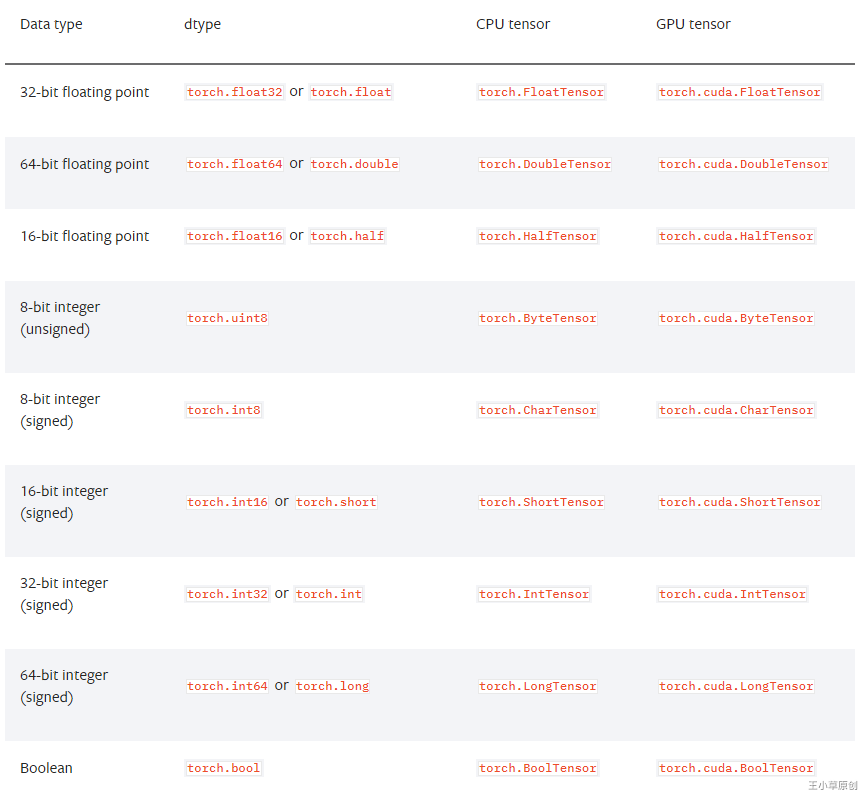# 2.3 Tensor类型

• 第二章：认识Tensor的类型、创建、存储、api等，打好Tensor的基础，是进行PyTorch深度学习实践的重中之重的基础。
• 第三章：学习PyTorch如何读入各种外部数据
• 第四章：利用PyTorch从头到尾创建、训练、评估一个模型，理解与熟悉PyTorch实现模型的每个步骤，用到的模块与方法。
• 第五章：学习如何利用PyTorch提供的3种方法去创建各种模型结构。
• 第六章：利用PyTorch实现简单与经典的模型全过程:简单二分类、手写字体识别、词向量的实现、自编码器实现。
• 第七章利用PyTorch实现复杂模型：翻译机（nlp领域）、生成对抗网络（GAN)、强化学习(RL)、风格迁移（cv领域）。
• 第八章：PyTorch的其他高级用法：模型在不同框架之间的迁移、可视化、多个GPU并行计算。（1）创建Tensor时用参数指明数据类型

import torch

double_points = torch.ones((10, 2), dtype=torch.double)
short_points = torch.tensor([[1,2],[3,4]], dtype=torch.short)


（2）获取tensor的数据类型

short_points.dtype

torch.int16


（3）转换tensor的数据类型

# （1）直接在tensor后面接.dtype()进行转换
double_points = torch.zeros(10,2).double()

# （2）使用to进行转换
double_points = torch.zeros(10,2).to(torch.double)

# （3）使用type()进行转换
double_points = torch.zeros(10,2).type(torch.short)


（4）设置/获取默认Tensor类型

# 指定
torch.set_default_tensor_type(torch.double)
# 获取
torch.get_default_tensor_type()